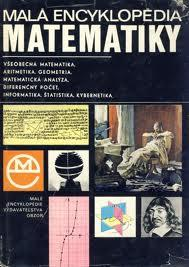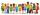# Book

To number pages of thick book was used 4201 digits. How many pages has this book?

Correct result:

x =  517

#### Solution:We would be pleased if you find an error in the word problem, spelling mistakes, or inaccuracies and send it to us. Thank you!Tips to related online calculators
Check out our ratio calculator.
Do you have a linear equation or system of equations and looking for its solution? Or do you have quadratic equation?

## Next similar math problems:

• Simple equationSolve for x: 3(x + 2) = x - 18
• Simple equation 1035= 7*3*x what is x?
• Forest nurseryIn the forest nursery after winter, they found that 1/10 stems died out of them. For them, they land 193 new spruces. How many spruces are in the forest nursery?
• Simple equation 8Solve the following equation: 36=-(1+7x)-6(-7-x)
• Equation 29Solve next equation: 2 ( 2x + 3 ) = 8 ( 1 - x) -5 ( x -2 )
• Find xSolve: if 2(x-1)=14, then x= (solve an equation with one unknown)
• Unknown number 11That number increased by three equals three times itself?
• Simple equationSolve the following simple equation: 2. (4x + 3) = 2-5. (1-x)
• Simple equation 9Solve the following equation: -8y+5=-9y+9
• NormThree workers planted 3555 seedlings of tomatoes in one dey. First worked at the standard norm, the second planted 120 seedlings more and the third 135 seedlings more than the first worker. How many seedlings were standard norm?
• Negative in equation2x + 3 + 7x = – 24, what is the value of x?
• The math testThe math test contains 20 problems. For each correctly solved problem, the solver gets 3 points, for each incorrectly solved or unsolved problem, 2 points are deducted. Ondrej got 25 points. How many problems did he solve correctly?
• Tickets 3A total of 645 tickets were sold for the school play. They were either adult tickets or student tickets. There were 55 fewer student tickets sold than adult tickets. How many adult tickets were sold?
• QuizTested student answered correctly on a 4/ 8 questions. Wrong answers was 16. How many questions answered?
• Dropped sheetsThree consecutive sheets dropped from the book. The sum of the numbers on the pages of the dropped sheets is 273. What number has the last page of the dropped sheets?
• InfirmaryTwo thirds of children from the infirmary went on a trip seventh went to bathe and 40 children remained in the gym. How many children were treated in the infirmary?
• The classroomIn the seventh class the number of boys and girls are at a ratio of 4:5. Boys are 16. How many total students are in the classroom?